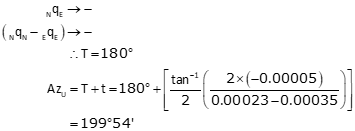## 3. Example

From the adjusted network of Chapter E Section 6, Figure G-13, determine the standard error ellipse and 95% CI for each adjusted point.Figure G-13 Adjusted Network

The network had three DF and a So of ±2.136'. The last covariance matrix was:### a.  Sub-Matrices### b. AzU of Each Error Ellipse

Point JPoint K### c. Rotated Covariance Matrices Diagonal Elements

Point JPoint K### d. Standard Error Ellipse Parameters

Point JPoint K### e. 95% CI Error Ellipse Parameters

Table G-2: At 3 DF, F Statistic = 9.55

Point JPoint K### f. SummaryPoint AzU a, SU b, SV 95% SU 95% SV J 215°04' ±0.046' ±0.021' ±0.201' ±0.092' K 199°54' ±0.041' ±0.031' ±0.179' ±0.135'